# C++ program to Reverse a Sentence Using Recursion

In this tutorial you will learn about the C++ program to Reverse a Sentence Using Recursion and its application with practical example.

## C++ program to Reverse a Sentence Using Recursion

In this tutorial, we will learn to create a C++ program that will C++ Program to Reverse a Sentence Using Recursion in C++ programming.

## Prerequisites

Before starting with this tutorial we assume that you are best aware of the following C++ programming topics:

• Operators in C++ Programming.
• Usage of Conditional statements in C++ Programming.
• Basic Input and Output function in C++ Programming.
• Basic C++ programming.

## What is Recursion? Reverse a Sentence Using it?

The recursion means a function or program that repeats itself until the condition is satisfied. The recursion repeats the program execution in a controlled format. Today we will reverse a sentence using the recursion.

## Reverse a Sentence Using Recursion

In this program of recursion, we will take the input sentence from the user and store it in the string variable. Then will find the length of that string and at last, we will reverse that string using a recursion program.

With the help of the below, code we will Reverse a Sentence Using Recursion.

## Output:-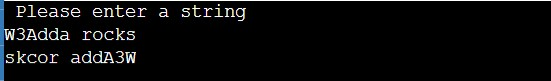In the above program, we have first initialized the required variable.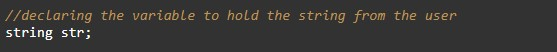• str = string type variable that will hold the value of the input sentence.

Input strings from the user for program.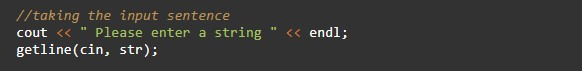Reverse printing function for the sentence.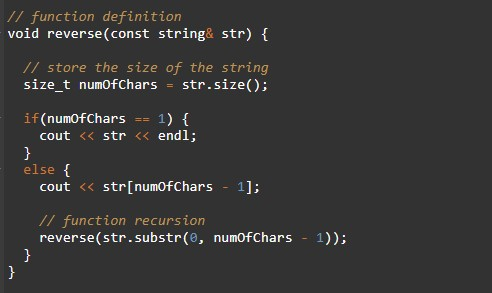Main Function of the program.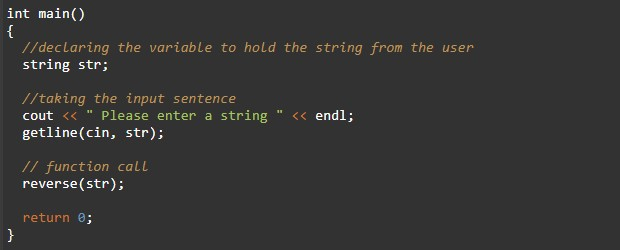In this tutorial we have learn about the C++ program to Reverse a Sentence Using Recursion and its application with practical example. I hope you will like this tutorial.